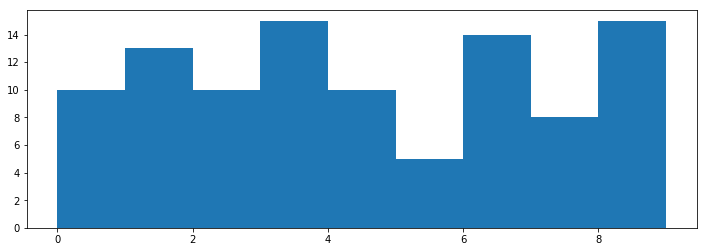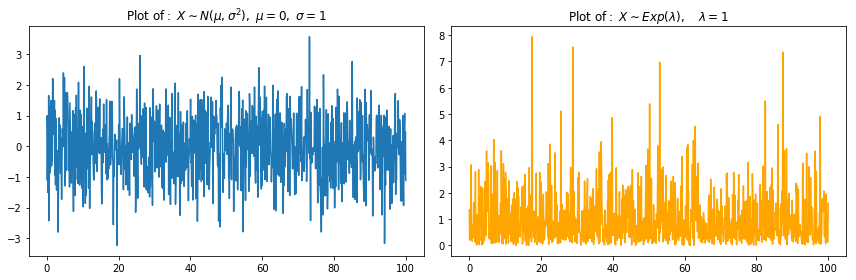# Introduction¶

You can select the box with Code and change it to Markdown in order to write Markdown code. Then press the Run button on the selected cell (or press Ctrl + Enter). You can press Enter to go to a new lien in your code block. This will not run your code and allow you to write multiple lines at once.

If you'd like to edit a cell - double click it.

You can have multiple code blocks of Markdown one after the other (note that the use of --- added a horizontal line - we used it as an example to show where the new code block started, you can also see which code belongs to the slected block hi the highlight on the left). This would allow you to section-off some parts of your text, if it gets pretty large.

Since we are using Markdown, we can use similar functionality we use in RStudio's RMarkdown - A quick comparison of Markdown and RMarkdown.

We can still use lists:

• One
• A
• B
• C
• Two
• Three

We can also use Latex for Math notation:

with $:$X_1, X_2, ..., X_3and with $$:$$Y_t = \sum_{j = 1}^t \epsilon_t^2$$As well as matrices:$$ \begin{bmatrix} \alpha& \beta^{*}\\ \gamma^{*}& \delta \end{bmatrix}, \quad \begin{pmatrix} \alpha& \beta^{*}\\ \gamma^{*}& \delta \end{pmatrix} Aligned equations: \begin{align} Y_{1,t} &= \alpha_1 + \beta_1 X_{1,t} + \epsilon_{1,t} \\ Y_{2,t} &= \alpha_1 + \beta_1 X_{1,t} + \epsilon_{1,t} \end{align} and \begin{align} Population_t &= \alpha_1 + \gamma_1 X_{1,t} + \gamma_2 X_{1,t-1} \\ Price_t &= \alpha_2 + \beta_1 Z_{1,t} \end{align} And equation systems: f(n) = \begin{cases} n/2 &\mbox{if } n \equiv 0 \\ (3n +1)/2 & \mbox{if } n \equiv 1. \end{cases} \pmod{2}$(sometimes the formulas might not generate at first run - double click the code cell and select Run to again evaluate the code) Another difference from RMarkdown in Rstudio is that we do not need to re-generate the whole document each time! # Python code¶ As mentioned, we can run Python code here as well by inserting a new code block with Code instead of Markdown selected. In : #Import the required modules import numpy as np np.random.seed(123) nsample = 1000 eps = np.random.normal(size=nsample) print(eps.mean())  -0.0395641360808  Note that we can re-run this code chunk to get the same average value. If we try to run the next block more than once, we will get different results, much like with Rmarkdon in R: In : eps = np.random.normal(size=nsample) print(eps.mean())  0.00838916739587  Note that, if we run the 1st block and then the 2nd block - we will get the same results as long as they are run in the same order only once. As with R, always set a seed before random number generation in the same block, in order to make sure that your results are reproducible! We can also define our functions in one code block: In : def my_add(x, y): x = x + 1 y = y + 1 return x + y  And use them in a different code block: In : print(my_add(1, 2))  5  We can also plot our data. # Plot of a histogram¶ In : np.random.seed(123) x = np.random.randint(10, size = (1, 100)) x_integer = x #print(np.arange(x.min(), x.max() + 1))  In : #If we want interactivity in our plots, uncomment one of the following before importing matplotlib: #%matplotlib inline #%matplotlib notebook #However, currently JavaScript output is disabled in JupyterLab so this will not work... import matplotlib.pyplot as plt plt.hist(x_integer, bins = np.arange(x_integer.min(), x_integer.max() + 1)) plt.show()# Multiple plots example¶ In : np.random.seed(123) x = np.linspace(0, 100, nsample) y1 = np.random.normal(size = nsample) y2 = np.random.exponential(size = nsample) print(y1.mean()) print(y2.mean())  -0.0395641360808 1.00729863478  In : #If we want to change our figure sizes: fig_size = [12, 4] plt.rcParams["figure.figsize"] = fig_size #a 1-row, 2-column figure: go to the first subplot plt.subplot(1, 2, 1) plt.plot(x, y1) plt.title(r'$\mathrm{Plot\ of:}\ X \sim N(\mu, \sigma^2),\ \mu=0,\ \sigma=1$') #a 1-row, 2-column figure: go to the second subplot. plt.subplot(1, 2, 2) plt.plot(x, y2, color = 'orange') plt.title(r'$\mathrm{Plot\ of:}\ X \sim Exp(\lambda),\quad \lambda=1\$')

#minimize the overlap of subplots (titles, axis labels etc.):
plt.tight_layout()
plt.show()